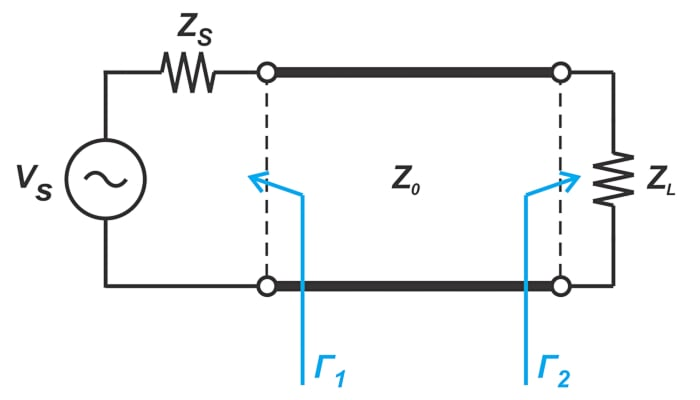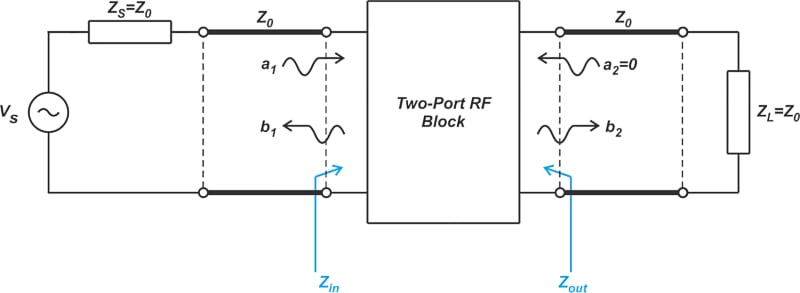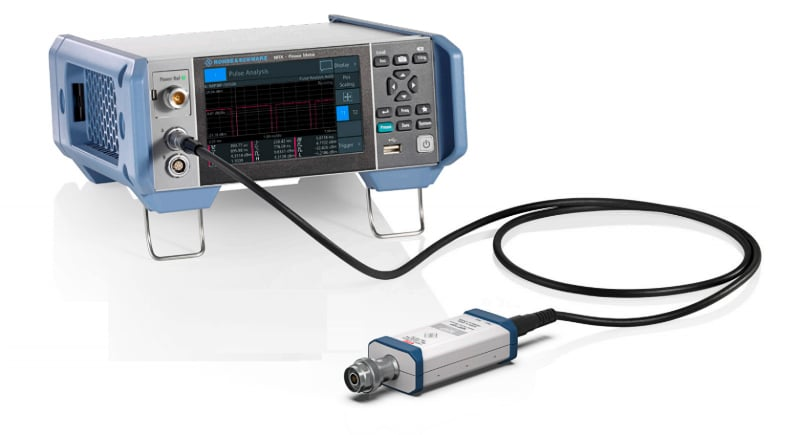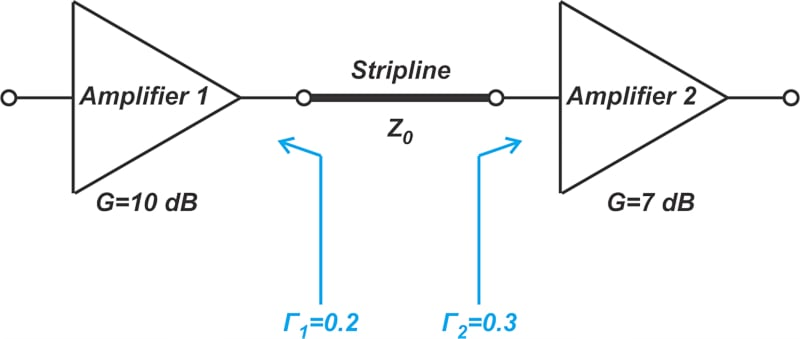Technical Article

# Mismatch Loss Effect on RF Power Measurement and Gain of Cascaded Amplifiers

February 15, 2023 by Dr. Steve Arar

## Using the mismatch loss equations, learn about the effect of mismatch loss on RF power measurement and the gain of cascaded amplifiers.

Effective power transfer is a major concern in an RF design. Since impedance discontinuities can reflect electrical waves, they can cause power loss, commonly referred to as mismatch loss (ML), which manifests itself in various applications. For example, the power measured by an RF power sensor, as well as the effective gain of a cascade of RF blocks, are affected by wave reflections. For a cascade of RF blocks, we aim to minimize the mismatch loss so that we can transfer as much power as possible. Additionally, by minimizing the mismatch loss and developing appropriate statistical models for this error, we can estimate the uncertainty in our systems.

In this article, we’ll first examine the mismatch loss equations. Then, we’ll discuss the effect of this phenomenon on RF power measurement and the effective gain of cascaded amplifiers.

### Mismatch Loss: Two Different Definitions

Consider the diagram in Figure 1 that shows a transmission line connected to mismatched impedances (Z≠ Z0 and Z≠ Z0) at both the input and output ports.##### Figure 1. Example diagram showing a transmission line connected to mismatched impedances at both input and output ports.

Equation 1 shows one way of defining the mismatch loss for the above circuit:

$ML = \frac{|1- \Gamma_1 \Gamma_2|^2}{\big ( 1-|\Gamma_1|^2 \big )\big ( 1-|\Gamma_2|^2 \big )}$

##### Equation 1.

This equation, which was examined in great detail in the previous article, gives the power loss with respect to the power available from the source. For example, if the power delivered by the source to a conjugate-matched load is -30 dBW and the ML is 1 dB for our actual load, then the power delivered to the load is -31 dBW.

With the above definition, the reference power is the power available from the source. It is common to define mismatch loss using another (actually more useful) reference power; the power the source delivers to a Z0 termination (where Z0 is the characteristic impedance of the line, with 50 Ω being a standard value).

With that in mind, you might wonder why the power that can be delivered to a Z0 termination is of interest to us. In RF systems, most circuits are designed assuming they will be used with some known characteristic impedance. In other words, during normal operation, most circuits are assumed to see a Z0 source resistance and a Z0 load resistance. That’s why RF blocks are usually characterized under these conditions. To better understand this functionality, consider the test setup to measure the S-parameters of a two-port network (Figure 2).##### Figure 2. Example diagram set up to measure S-parameters of a two-port network.

For S-parameter measurements, one port is driven with a source whose series resistance is Z0, and the other port is terminated with a Z0 load. Using the above diagram, we can measure the input reflection coefficient (S11) and the transmission coefficient from port 1 to port 2 (S21).

Note that a Z0 termination at the output port ensures that no energy reflects off of the load (a= 0) and, thus, b1 and b2 are only produced as a result of the traveling wave that is incident on the input port (a1). It’s also worth mentioning that the network output impedance Zout doesn’t have to be equal to Z0. In fact, it is rare that Zout = Z0. We only need to have Z= Z0 to ensure that a= 0. By definition, S-parameters are based on a test setup that uses matched terminations. This significantly simplifies the measurement of the S-parameters compared to other types of two-port network representations, such as T-parameters.

Since the response of RF blocks is normally characterized in a Z0 environment (Z= Z= Z0 with Z= 50 Ω being a standard value), it is desired to find the mismatch loss with respect to the power that a source delivers to a Z0 termination.

With the circuit in Figure 1, the general term “matched load” can refer to two different conditions: $$Z_L=Z_S^*$$ and Z= Z0. The first condition corresponds to the maximum power transfer theorem, whereas the second condition gives a reflectionless load. The use of the term matched load can sometimes cause confusion. To be more clear, we can use the term “conjugate match” to refer to $$Z_L=Z_S^*$$ and the terms “Z0 match” or “reflectionless match” to describe Z= Z0.

### Mismatch Loss WRT Power Delivered to Z0 Termination

Considering the diagram in Figure 1, it can be shown that the mismatch loss (ML) with respect to the maximum power that can be delivered to a load impedance of Z0 is given by:

$ML = \frac{|1- \Gamma_1 \Gamma_2|^2}{ 1-|\Gamma_2|^2 }$

##### Equation 2.

Note that Γ1 and Γ2 denote the reflection coefficient at the source and load end of the line, respectively. With ML defined as shown in Equation 2, the power delivered to a Z0 termination (PZ0) and the power delivered to an arbitrary load (PLoad) are related by the following equation:

$P_{Load}=\frac{P_{Z0}}{ML}$

##### Equation 3.

We can also express the above equation in decibels. In many applications, the phase angle of Γ1 and Γ2 is not known; and we can find only the upper and lower bound of ML to determine the range of power transfer uncertainty. The difference between maximum and minimum values of ML, which is known as mismatch uncertainty (MU), is given by:

$\begin{eqnarray} MU &=& 20log(ML_{max})-20log(ML_{min})\\ &=& 20log \big ( 1+ | \Gamma_1 \Gamma_2| \big ) - 20log \big ( 1 - | \Gamma_1 \Gamma_2| \big ) \end{eqnarray}$

##### Equation 4.

In the previous article, we derived this same equation using Equation 1 rather than Equation 2. Although Equations 1 and 2 give the power loss with respect to two different reference powers, they lead to the same mismatch uncertainty term, as expected. Let’s look at an example to see how the above equations are used in a power sensor application.

### Example 1: RF Power Sensor

As the name clearly reveals, a power sensor is used to measure the power of RF and microwave signals (Figure 3).##### Figure 3. R&S NRX power meter connected to a pulsed power sensor. Image used courtesy of Rohde & Schwarz

Ideally, the sensor should measure the net power delivered to the sensor. This is not the case in practice because some of the net input power might not dissipate in the sensing element. For example, losses due to radiation might guide the energy away from the sensing element. Therefore, the power that is ultimately metered and indicated by the sensor Pm is not exactly the same as the net power delivered to the sensor PLoad. Test equipment manufacturers use some calibration coefficients to describe the relationship between these two quantities:

$P_m = \eta _{e}P_{Load}$

##### Equation 4.

In the above equation, ηe is called “effective efficiency.” When characterizing a generator, the desired quantity is usually the power that would be dissipated in a Z0 load rather than that dissipated in the input impedance of the power sensor. Substituting Equations 2 and 3 in Equation 4 produces an equation for PZ0:

$\begin{eqnarray} P_m &=& \eta _{e}\frac{P_{Z0}}{ML}\\ &=& P_{Z0}\times \eta _{e} \big ( 1-|\Gamma_2|^2 \big ) \times \frac{1}{|1- \Gamma_1 \Gamma_2|^2} \end{eqnarray}$

##### Equation 5.

The factor $$\eta _e \big (1-|\Gamma_2|^2)$$ is called the calibration factor Kb. Most modern power meters have the ability to trim out the error from the calibration factor. When this feature is used, Equation 5 can be rewritten as:

$P_{Z0} = P_{m}\times {|1- \Gamma_1 \Gamma_2|^2}$

Note that the error term is actually related to the mismatch uncertainty (MU) discussed above. For example, if $$| \Gamma_1 |$$ ≤ 0.09 and $$| \Gamma_2 |$$ ≤ 0.2, the maximum and minimum of the error are:

$\begin{eqnarray} Error_{Max} &=& {|1+ \Gamma_1 \Gamma_2|^2} \\ &=& \big ( 1+0.09 \times 0.2 \big ) ^2 \\ &=&1.036=0.15 \text{ } dB \end{eqnarray}$

and

$\begin{eqnarray} Error_{Min} &=& {|1- \Gamma_1 \Gamma_2|^2} \\ &=& \big ( 1-0.09 \times 0.2 \big ) ^2 \\ &=&0.964=-0.16 \text{ } dB \end{eqnarray}$

Therefore, the actual value of PZ0 can be 0.15 dB higher or 0.16 dB lower than the value indicated by the power meter.

### Example 2: Effective Gain of Cascaded Blocks

Consider the configuration shown in Figure 4.##### Figure 4. Example configuration of amplifiers connected via a stripline.

In this example, the power gain of amplifiers 1 and 2 is 10 dB and 7 dB, respectively. Due to impedance mismatch at the two ends of the stripline, part of the energy provided by Amplifier 1 bounces back and forth between the two impedance discontinuities. It can be shown that these wave reflections lead to a power loss given by:

$ML= 20log(1 \pm |\Gamma_1||\Gamma_2| )$

##### Equation 6.

You can find the proof of this equation in Chapter 2 of Practical RF System Design by W. F. Egan. For example, if $$| \Gamma_1 |$$ ≤ 0.2 and $$| \Gamma_2 |$$ ≤ 0.3, the maximum and minimum of the mismatch-induced loss are 0.51 dB and -0.54 dB, respectively. A negative loss of 0.54 dB actually represents an additional power gain. Now we can find the effective gain of the cascade. Normally, we expect the above circuit to have a gain of 10+7 = 17 dB; however, because of the mismatch loss, the actual gain can vary between 17 - 0.51 = 16.49 dB and 17 + 0.54 = 17.54 dB.

### Mismatch Loss and Impedance in RF Power Transfer Design

Impedance discontinuities prevent us from having an effective power transfer in an RF design. This manifests itself as power loss and leads to uncertainty in various applications. In this article, we discussed that the power measured by an RF power sensor and the effective gain of cascaded amplifiers are affected by the mismatch loss. In the next article, we’ll discuss the cascade gain in greater detail and look at methods of reducing the mismatch uncertainty.